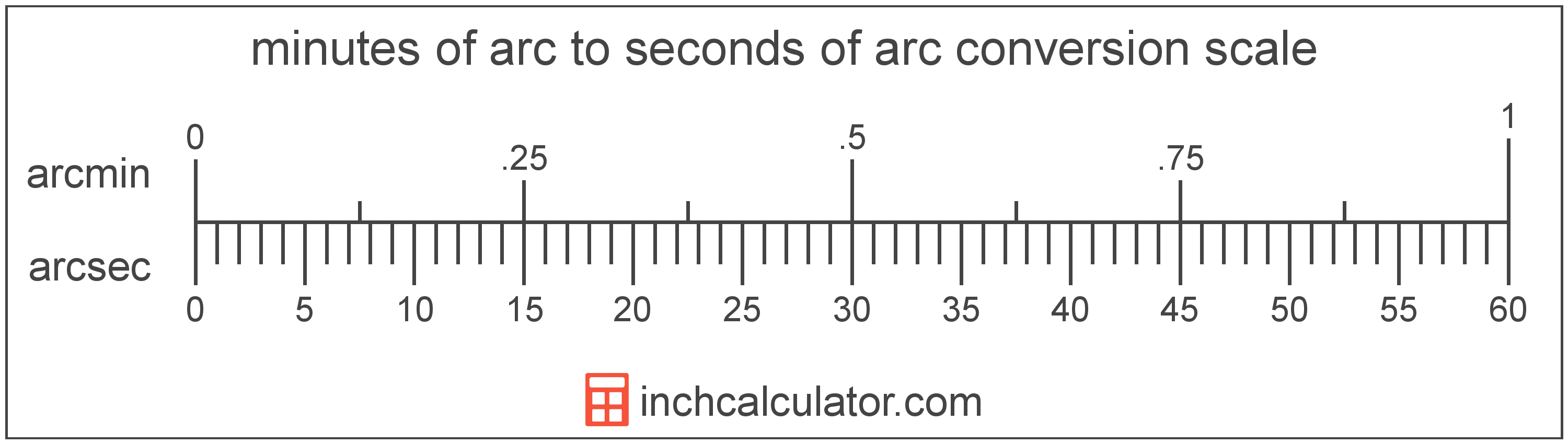# Seconds Of Arc to Minutes Of Arc Conversion

Enter the angle in seconds of arc below to get the value converted to minutes of arc.

Results in Minutes Of Arc:1 arcsec = 0.016667 arcmin

## How to Convert Seconds Of Arc to Minutes Of ArcTo convert a second of arc measurement to a minute of arc measurement, divide the angle by the conversion ratio.

Since one minute of arc is equal to 60 seconds of arc, you can use this simple formula to convert:

minutes of arc = seconds of arc ÷ 60

The angle in minutes of arc is equal to the seconds of arc divided by 60.

For example, here's how to convert 5 seconds of arc to minutes of arc using the formula above.
5" = (5 ÷ 60) = 0.083333'

Seconds of arc and minutes of arc are both units used to measure angle. Keep reading to learn more about each unit of measure.

## Seconds Of Arc

The second of arc is a unit of angle equal to 1/60th of one minute of arc or 1/3,600 of one degree.

A second of arc is sometimes also referred to as an arc second or arcsecond. Seconds of arc can be abbreviated as arcsec, and are also sometimes abbreviated as asec. For example, 1 second of arc can be written as 1 arcsec or 1 asec.

The second of arc is most commonly expressed using a double prime (″), though a double quote is often used as well. For example, 1 second of arc is most often written as 1″.

## Minutes Of Arc

The minute of arc is a unit of angle equal to 1/60th of one degree, or 1/21,600 of a circle. The minute of arc is also equal to π/10,800 radians.

A minute of arc is sometimes also referred to as an arc minute, arcminute, or minute arc. Minutes of arc can be abbreviated as arcmin, and are also sometimes abbreviated as MOA or amin. For example, 1 minute of arc can be written as 1 arcmin, 1 MOA, or 1 amin.

The minute of arc is most commonly represented using the prime (′), although the single-quote is commonly used. For instance, 1 minute of is most commonly expressed as 1′.

## Second Of Arc to Minute Of Arc Conversion Table

Second of arc measurements converted to minutes of arc
Seconds Of Arc Minutes Of Arc
1" 0.016667'
2" 0.033333'
3" 0.05'
4" 0.066667'
5" 0.083333'
6" 0.1'
7" 0.116667'
8" 0.133333'
9" 0.15'
10" 0.166667'
11" 0.183333'
12" 0.2'
13" 0.216667'
14" 0.233333'
15" 0.25'
16" 0.266667'
17" 0.283333'
18" 0.3'
19" 0.316667'
20" 0.333333'
21" 0.35'
22" 0.366667'
23" 0.383333'
24" 0.4'
25" 0.416667'
26" 0.433333'
27" 0.45'
28" 0.466667'
29" 0.483333'
30" 0.5'
31" 0.516667'
32" 0.533333'
33" 0.55'
34" 0.566667'
35" 0.583333'
36" 0.6'
37" 0.616667'
38" 0.633333'
39" 0.65'
40" 0.666667'